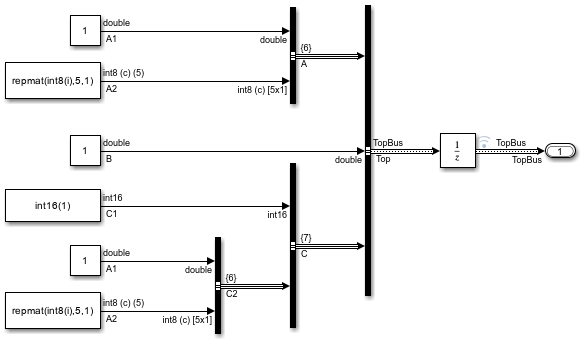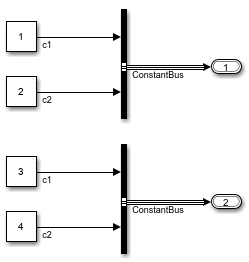Create MATLAB structures that use the same hierarchy and attributes as buses

## Syntax

``structs = Simulink.Bus.createMATLABStruct(buses)``
``structs = Simulink.Bus.createMATLABStruct(buses,values)``
``structs = Simulink.Bus.createMATLABStruct(buses,values,dims)``
``structs = Simulink.Bus.createMATLABStruct(buses,values,dims,scope)``

## Description

example

````structs = Simulink.Bus.createMATLABStruct(buses)` creates one or more MATLAB® structures that have the same hierarchy and attributes as the specified buses. The resulting structures use the ground values of the buses. Use this syntax to create initialization structures for multiple bus ports.```

example

````structs = Simulink.Bus.createMATLABStruct(buses,values)` creates one or more structures that use the specified values.```

example

````structs = Simulink.Bus.createMATLABStruct(buses,values,dims)` creates one or more structures that have the specified dimensions. To create a structure for an array of buses, include the `dims` argument.```
````structs = Simulink.Bus.createMATLABStruct(buses,values,dims,scope)` creates one or more structures in the data dictionary specified by `scope`.```

## Examples

collapse all

Open and simulate model `ex_bus_initial_conditions`.

```open_system('ex_bus_initial_conditions') sim('ex_bus_initial_conditions'); ```Create a MATLAB structure using bus object `Top`, which model `ex_bus_initial_conditions` loads.

```mStruct = Simulink.Bus.createMATLABStruct('Top'); ```

Set a value for the field of the `mStruct` structure that corresponds to bus element `A1` of bus `A`.

```mStruct.A.A1 = 3; mStruct.A ```
```ans = struct with fields: A1: 3 A2: [5x1 int8] ```

Simulink sets the other fields in the structure to the ground values of the corresponding bus elements.

You can use `mStruct` as the initial condition structure for the Unit Delay block.

Create a MATLAB structure for a bus whose signal elements use a data type other than `double`. Use a partial structure to specify initialization values for a subset of the elements. When you create the partial structure, match the data types of the fields with the data types of the corresponding elements.

Open and simulate model `ex_bus_initial_conditions`.

```open_system('ex_bus_initial_conditions') sim('ex_bus_initial_conditions'); ```The `C1` signal element that the block labeled `Constant5` produces uses the data type `int16`.

Find the port handle for the Bus Creator block port that produces the `Top` bus signal.

```ph = get_param('ex_bus_initial_conditions/TopBus','PortHandles'); ```

Create a partial structure that specifies values for a subset of the elements in the bus signal created by the `TopBus` block. To set the value of the `C.C1` field, use a typed expression. Match the data type in the expression with the data type of the signal element in the model (`int16`).

```PartialstructForK = struct('B',3,'C',struct('C1',int16(5))); ```

Create a full structure by using the port handle (`ph`) for the `TopBus` block. Override the ground values for the `C.C1` and `B` elements.

```outPort = ph.Outport; mStruct = Simulink.Bus.createMATLABStruct(outPort,PartialstructForK); ```

The field `C.C1` in the output structure continues to use the data type `int16`.

Open and simulate model `ex_bus_initial_conditions`.

```open_system('ex_bus_initial_conditions') sim('ex_bus_initial_conditions'); ```Create a partial structure for a subset of bus elements in the bus created by the `TopBus` block.

```PartialStructForK = struct('A',struct('A1',4),'B',3) ```
```PartialStructForK = struct with fields: A: [1x1 struct] B: 3 ```

Create a MATLAB structure using bus object `Top`, a partial structure, and dimensions for the resulting structure.

```structFromBus = Simulink.Bus.createMATLABStruct... ('Top',PartialStructForK,[2 3]) ```
```structFromBus = 2x3 struct array with fields: A B C ```

To create initialization structures for multiple bus ports, specify port handles as arguments for `Simulink.Bus.createMATLABStruct`. The resulting cell array of structures uses ground values.

Open and simulate model `ex_two_outports_create_struct`.

```open_system('ex_two_outports_create_struct') sim('ex_two_outports_create_struct'); ```Find the port handles for the Bus Creator blocks `Bus1` and `Bus2`.

```ph_1 = get_param... ('ex_two_outports_create_struct/Bus Creator','PortHandles'); ph_2 = get_param... ('ex_two_outports_create_struct/Bus Creator1','PortHandles'); ```

Create a MATLAB® structure using an array of port handles.

```mStruct = Simulink.Bus.createMATLABStruct([ph_1.Outport ph_2.Outport]) ```
```mStruct = 2x1 cell array {1x1 struct} {1x1 struct} ```

Create a MATLAB structure based on a port that connects to a bus signal. Use a partial structure to specify values for a subset of the bus elements in the bus that connects to the port.

Open and simulate model `ex_bus_initial_conditions`.

```open_system('ex_bus_initial_conditions') sim('ex_bus_initial_conditions'); ```Find the port handle for the Bus Creator block port that produces the `Top` bus signal. The `Outport` handle is the handle that you need.

```ph = get_param('ex_bus_initial_conditions/TopBus','PortHandles') ```
```ph = struct with fields: Inport: [31.0012 29.0012 41.0012] Outport: 42.0012 Enable: [] Trigger: [] State: [] LConn: [] RConn: [] Ifaction: [] Reset: [] ```

Create a partial structure for the bus signal created by the `TopBus` block. You can use a partial structure to specify values for a subset of bus elements.

```PartialstructForK = struct('A',struct('A1',4),'B',3) ```
```PartialstructForK = struct with fields: A: [1x1 struct] B: 3 ```

Bus elements represented by structure fields `Top.B` and `Top.A` are at the same level in the bus hierarchy. You can use this partial structure to override the ground values for the `B` and `A` bus signal elements.

When you create a structure from a bus object or from a bus port, you can use a partial structure as an optional argument.

Create a MATLAB structure by using the port handle (`ph`) for the `TopBus` block. Override the ground values for the `A.A1` and `B` bus elements.

```outPort = ph.Outport; mStruct = Simulink.Bus.createMATLABStruct(outPort,PartialstructForK) ```
```mStruct = struct with fields: A: [1x1 struct] B: 3 C: [1x1 struct] ```

## Input Arguments

collapse all

Source of the bus information, specified as a `Bus` object name, port handle, cell array of `Bus` object names, or array of port handles.

• If you use a `Bus` object name, then the `Bus` object must be in the MATLAB base workspace or the data dictionary used by the model. The data type for a `Bus` object name is `char` or `string`.

• If you use a port handle, then the model must compile successfully before you use this function. The data type for a port handle is `double`.

• For an array of buses, you cannot use a port handle.

• If you use the `dims` argument, then for the `buses` argument, use a `Bus` object or cell array of `Bus` objects.

Specifying a cell array of `Bus` object names or an array of port handles creates multiple structures with one `Simulink.Bus.createMATLABStruct` call and provides better performance than using separate `Simulink.Bus.createMATLABStruct` calls to create the structures.

Example: ```struct = Simulink.Bus.createMATLABStruct('BusObject')```

Example: ```structs = Simulink.Bus.createMATLABStruct({'BusObject','BusObject1'})```

Example: ```struct = Simulink.Bus.createMATLABStruct(portHandle)```

Example: ```structs = Simulink.Bus.createMATLABStruct([portHandle,portHandle1])```

Data Types: `double` | `char` | `string` | `struct` | `cell`

Values for a subset of elements in the resulting structure, specified as an empty matrix (`[]`), partial structure, or cell array. The cell array must contain a partial structure or empty matrix for each specified source of bus information.

For information on creating partial structures, see Create Partial Structures for Initialization.

To use ground values, use an empty matrix.

Example: ```struct = Simulink.Bus.createMATLABStruct('BusObject',PartialStruct) ```

Data Types: `struct` | `cell`

Dimensions of the resulting structure, specified as a vector.

Each dimension element must be an integer that is greater than or equal to 1. If you specify a partial structure for the `values` argument, each dimension element must be greater than or equal to its corresponding dimension element in the partial structure.

Example: ```struct = Simulink.Bus.createMATLABStruct('BusObject',PartialStruct,[2 3]) ```

Example: ```structs = Simulink.Bus.createMATLABStruct({'Bus','Bus1','Bus2'},{[],[],[]},{1,2,3})```

Data Types: `double` | `cell`

Data dictionary, specified as a `Simulink.data.Dictionary` object. Before you use this argument, represent the dictionary with a `Simulink.data.Dictionary` object by using, for example, the `Simulink.data.dictionary.create` or `Simulink.data.dictionary.open` function.

If `scope` is empty, the function uses the MATLAB base workspace as the source of the `Bus` objects.

Example: ```structs = Simulink.Bus.createMATLABStruct({'Bus','Bus1','Bus2'},{[],[],[]},{1,1,1},dataDictionaryObject)```

## Output Arguments

collapse all

Structures with the same signal hierarchy and attributes as buses, returned as a MATLAB structure or a cell array of MATLAB structures.

The structure dimensions depend on the input arguments you specify:

• If you specify only the `buses` argument, then the dimension is 1.

• If you also specify the `values` argument, then the dimensions match the dimensions of `values`.

• If you specify the `dims` argument, then the dimensions match the dimensions of `dims`.

## Tips

• If you use the `Simulink.Bus.createMATLABStruct` function repeatedly for the same model (for example, in a loop in a script), you can improve performance by avoiding multiple model compilations. For improved speed, put the model in compile before using the function multiple times. For example, to put the `vdp` model in compile, use this command:

`vdp([],[],[],'compile')`

After you create the MATLAB structure, terminate the compile by using this command:

`vdp([],[],[],'term')`
• You can use the Bus Editor to invoke the `Simulink.Bus.createMATLABStruct` function by using one of these approaches:

• Select the File > Create a MATLAB structure menu item.

• Select the `Bus` object for which you want to create a full MATLAB structure. Then, in the toolbar, click the button.

You can then edit the MATLAB structure in the MATLAB Editor and evaluate the code to create or update the values in this structure.

• You can use the `Simulink.Bus.createMATLABStruct` function to specify the initial value of the output of a referenced model. For details, see the “Referenced Model: Setting Initial Value for Bus Output” section of the Detailed Workflow for Managing Data with Model Reference example.

Introduced in R2010a